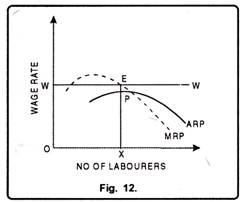Under perfect competition, equilibrium wage rate is determined where demand for labour is equal to supply of labour.

In other words, under perfect competition, a labourer will get wage equal to its marginal revenue productivity in the long run.

In Fig. 9 units of labour have been measured on X-axis and wages on Y-axis. DD and SS are the demand and Supply curves of labourers respectively. Both the curves intersect each other at point E which determines wage rate OP in the market.At this level of wages, ON units of labourers will get employment. Now suppose, wages go up to OP1. At this price, demand is ON1 and supply ON2. Since, the supply is more than demand; it will lead to competition among labourers to get employment which in turn results in a decrease in wage rate. On the other hand, if w age rate falls to OP2, demand will be more than the supply. This results in competition among the producers to get the services of labour which in turn leads to an increase in wage rate. Therefore, we may observe that equilibrium will be restored at that point where demand curve of labourers intersects the supply curve of labourers.

### Time Periods to Study Firm’s Equilibrium:

The study of firm’s equilibrium can be studied in the following two time periods:

1. Short Run

2. Long Run

#### 1. Short Run Period:

Short run refers to a period in which it is not possible for a firm to fully equate the demand for and supply of a factor. Therefore, a firm in the short run may face three situations.

Supernormal Profit:

If at OW wage rate, a firm provides employment to as much labourers whose ARP is more than the prevailing wage, the firm earns supernormal profits. In the Fig. 10 a firm at its equilibrium provides employment to OX labourers. Here ARP i.e. PX is greater than the wage rate EX. Thus, firm earns EP as supernormal profits.Normal Profit:

A firm enjoys normal profits, if ARP is equal to wage rate. In Fig. 11 when firm employs ON number of labourers, their ARP is EX which is equal to prevailing wage rate OW. Thus, the firm gets only normal profits.

A firm incurs losses, if at prevailing wage; a firm employs the number of labourers at which their ARP is less than the prevailing wage rate. In Fig. 12 when firm employs OX labourers, their ARP = PX is less than their wage rate OW. Thus, the firm has to incur losses equal to EP.

####2. Long Run Period:

In the long run, a firm earns normal profit. A firm will be in equilibrium where ARP is equal to MRP. In Fig. 13 when firm employs OX labourer ARP is equal to MRP.### Wage Determination under Imperfect Competition:

In the world of reality there exists imperfect competition rather than perfect competition. Therefore, Mrs. Joan Robinson and Prof. Pigou gave the wage rate determination under the conditions of imperfect competition.

The wage rate determination can be explained fewer than two heads:

(a) Perfect competition in product Market and Monopsony in the Labour market.

(b) Monopoly in the product Market and Monopsony in Labour Market.

#### (a) Perfect Competition in Product Market and Monopsony in Labour Market:

When there is a single buyer of labour in the market, monopsony is said to exist in the labour market. If there is an increase in monopolist’s demand for labour, wage rate will follow the same path which in turn tends to increase the average and marginal wage rate. It can be shown with the help of a diagram.

In Fig. 14 units of labour have been measured on X-axis while wages on Y-axis. ARP and MRP is the average revenue product and marginal revenue product curves. AW and MW are the upward sloping average wage and marginal wage curves indicating that if the monopolist wishes to employ more and more labourers, he has to offer the higher wage rate.The monopolistic firm is in equilibrium at point E. At point E both the conditions of equilibrium are fulfilled i.e. marginal wage should be equal to marginal revenue product and the marginal revenue product curve must cut the marginal wage curve from above and then lies below it. Thus, at this equilibrium level, he will employ OX units of labour and OP wage rate will be determined.

Since wages are less than marginal revenue productivity, means that the monopolist exploits the labour. Thus, in this equilibrium the monopolist earns the supernormal profits equal to the area PLMN. Therefore, it can be concluded that imperfect competition in the labour market results in the exploitation of labour.

#### (b) Monopoly in Product Market Monopsony in Labour Market:

When there exists monopoly in product market and monopsony in labour market then there is difference between marginal revenue product and value of marginal product. Value of marginal product refers to the product of MPP and the price of the commodity. It can be explained with the help of a figure.In Fig. 15 MRP is the marginal revenue product curve and VMP is the value of marginal product curve. The VMP curve is above the MRP curve. The monopsonist is in equilibrium at point E. The monopsonist employs ON units of labour and wage rate OP is determined in the market.